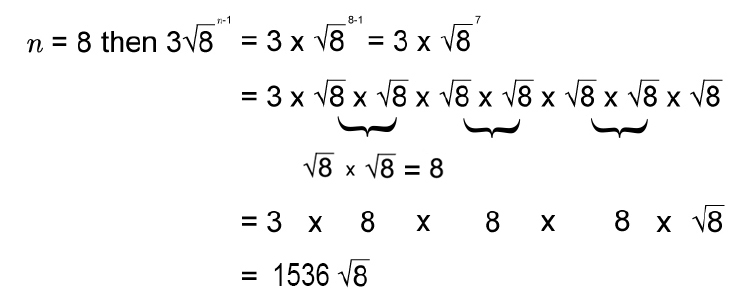# Formula for n^(th) term of a sequence - multiplication

In order to predict the n^(th) term of a sequence you will need to create a formula.

For sequence patterns of geometric progressions or geometric sequences (or multiplications) this is worked out by using the formula.

ar^(n-1)

Where

a= first term

r= the multiple

n=n^(th) numberArrrr (ar) not one (n-1) of us

ar^(n-1)

Example 1

We know the following sequence is a geometric sequence but what is the formula of the n^(th) term and what is the 8^(th) term.

 2 6 18 54 162 We can check that it is a multiple sequence by dividing each term by the previous term. 6/2=3 18/6=3 54/18=3 162/54=3

We have a multiple of 3

So the formula for this is

ar^(n-1)

So here

a= first term =2

r= the multiple =3

So the formula is

2times3^(n-1)

Now we need to check the formula is correct.

Try different values of n in the formula 2times3^(n-1)

n=1       term =2times3^(1-1)=2times3^0=2times1=2

n=2       term =2times3^(2-1)=2times3^1=2times3=6

n=3       term =2times3^(3-1)=2times3^2=2times9=18

n=4       term =2times3^(4-1)=2times3^3=2times27=54

This is correct

The 8th term would be

If n=8    then 2times3^(n-1)=2times3^(8-1)=2times3^7=4374

The 8th term =4,374

Example 2

We know the following sequence is a geometric sequence but what is the formula of the n^(th) term and what is the 8^(th) term.

 -2 4 -8 16 -32 64 We can check that it is a multiple sequence by dividing each term by the previous term. 4/-2=-2 (-8)/4=-2 16/-8=-2 (-32)/16=-2 64/-32=-2

We have a multiple of -2

So the formula for this is

ar^(n-1)

So here

a= first term =-2

r= the multiple =-2

So the formula is

-2times(-2)^(n-1)

Now we need to check the formula is correct.

Try different values of n in the formula -2times(-2)^(n-1)

n=1       term =-2times(-2)^(1-1)=-2times(-2)^0=-2times1=-2

n=2       term =-2times(-2)^(2-1)=-2times(-2)^1=-2times-2=4

n=3       term =-2times(-2)^(3-1)=-2times(-2)^2=-2times4=-8

n=4       term =-2times(-2)^(4-1)=-2times(-2)^3=-2times(-8)=16

This is correct

The 8th term would be

If n=8    then -2times(-2)^(n-1)=-2times(-2)^(8-1)=-2times(-2)^7

=-2times(-2xx-2xx-2xx-2xx-2xx-2xx-2)

=-2times(-128)=256

Answer =256

Example 3

We know the following sequence is a geometric sequence but what is the formula for the n^(th) term and what is the 8^(th) term.

 40 20 10 5 2.5 We can check that it is a multiple sequence by dividing each term by the previous term. 20/40=0.5 10/20=0.5 5/10=0.5 2.5/5=0.5

We have a multiple of 0.5

So the formula for this is

ar^(n-1)

So here

a= first term =40

r= the multiple =0.5

So the formula is

40times(0.5)^(n-1)

Now we need to check the formula is correct.

Try different values of n in the formula 40times(0.5)^(n-1)

n=1       term =40times(0.5)^(1-1)=40times0.5^0=40times1=40

n=2       term =40times(0.5)^(2-1)=40times0.5^1=40times0.5=20

n=3       term =40times(0.5)^(3-1)=40times0.5^2=40times0.5xx0.5=10

n=4       term =40times(0.5)^(4-1)=40times0.5^3=40times0.5xx0.5xx0.5=5

This is correct

The 8th term would be

If n=8    then 40times(0.5)^(n-1)=40times(0.5)^(8-1)=40times0.5^7

=40times(0.5xx0.5xx0.5xx0.5xx0.5xx0.5xx0.5)

=0.3125

Answer the 8th term =0.3125

Example 4

We know the following sequence is a geometric sequence but what is the formula of the n^(th) term and what is the 8^(th) term.

 3 3sqrt8 24 24sqrt8

We change to

 3 3sqrt8 3sqrt8sqrt8 3sqrt8sqrt8sqrt8 We can check that it is a multiple sequence by dividing each term by the previous term. (3sqrt8)/3=sqrt8 (3sqrt8sqrt8)/(3sqrt8)=sqrt8 (3sqrt8sqrt8sqrt8)/(3sqrt8sqrt8)=sqrt8

We have a multiple of sqrt8

So the formula for this is

ar^(n-1)

So here

a= first term =3

r= the multiple =sqrt8

So the formula is

3sqrt8^(n-1)

Now we need to check the formula is correct.

Try different values of n in the formula 3sqrt8^(n-1)

n=1       term =3sqrt8^(1-1)=3sqrt8^0=3times1=3

n=2       term =3sqrt8^(2-1)=3sqrt8^1=3sqrt8

n=3       term =3sqrt8^(3-1)=3sqrt8^2=3sqrt8sqrt8

n=4       term =3sqrt8^(4-1)=3sqrt8^3=3sqrt8sqrt8sqrt8

This is correct

The 8th term would beAnswer the 8th term =1536sqrt8